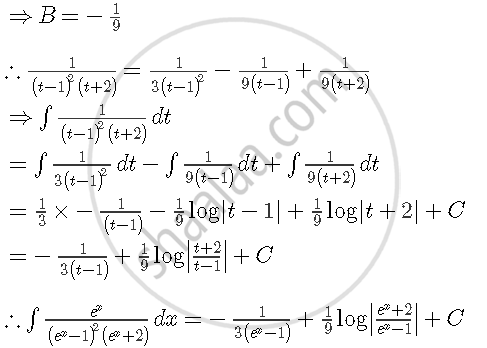Share

# Find Integral(E^X Dx)By((E^X - 1)Square2 (Ex + 2)) - CBSE (Science) Class 12 - Mathematics

ConceptMethods of Integration - Integration Using Partial Fractions

#### Question

Find int(e^x dx)/((e^x - 1)^2 (e^x + 2))

#### Solution

int(e^x dx)/((e^x - 1)^2 (e^x + 2))

Putting ex = t and exdx = dt, we get

int(e^x dx)/((e^x - 1)^2 (e^x + 2)) = int (dt)/((t-1)^2(t+2))

Using partial fraction, we have

1/((t-1)^2 (t + 1)) = A/(t-1)^2 +  B/(t -1) + C/(t +2)

⇒ 1 = A(t+2) + B(t−1)(t+2) + C(t−1)2 .....(1)

Putting t = 1 in (1), we get

A = 1/3

Putting t = −2 in (1), we get

C = 1/9

Comparing the coefficients of t2 on both sides of (1), we get

0Is there an error in this question or solution?

#### Video TutorialsVIEW ALL 

Solution Find Integral(E^X Dx)By((E^X - 1)Square2 (Ex + 2)) Concept: Methods of Integration - Integration Using Partial Fractions.
S